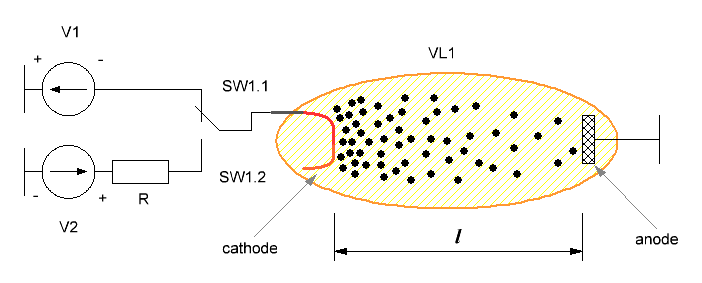Research website of Vyacheslav Gorchilin
2016-08-04
Tube method for increasing the efficiency of the second kind
Method is available for joint patenting
This method is based on the movement of electrons in a vacuum in a constant electric field. In this field the charges create an electronic cloud, the direction of which can be changed periodically, thereby increasing the efficiency of the second kind — $$\eta_2$$. In another way, this approach can be called the utilisation method of the electronic plasma. This principle reflects the following figure which shows:
• VL1 — vacuum tube with anode and cathode, the distance between which is equal to $$l$$;
• SW1 — switch with two positions S1.1 and SW1.2;
• R — resistance;
• V1, V2 voltage source. V1 is the voltage of the lamp during the live broadcast, V2 is the voltage in reverse direction.The device is quite simple. The first time the closed switch SW1.1 and a negative voltage across R1 is fed to the cathode of the lamp. With the cathodes break electrons and accelerating with an acceleration of $$a_1$$ reaches the anode. As soon as the first of them flew to the anode the switch moves to position S1.2 and to the cathode relative to the anode, served plus. Between the anode and cathode occurs a reverse electric field and charges begin retrograde motion, passing this time through the resistance $$R$$. Electron acceleration in this time can also be another, denote it by $$a_2$$.
The increase in $$\eta_2$$ denote koeffitsientom $$K_{\eta2}$$ and find it so: $K_{\eta2} = {W_R \over W_1 + W_2}$ where: $$W_R$$ is the energy passing through $$R$$, $$W_1$$ — energy expended in moving charges in the period closed SW1.1, $$W_2$$ is the energy expended for the return movement of charges in the period closed SW1.2. $$K_{\eta2}$$ has a complex relationship, in the most simple form it can be expressed as: $K_{\eta2} = 3{I_2 \, R \over V_1 + V_2 - I_2 \, R }$ where: $$I_2$$ is the current through $$R$$ in the second period of time, when SW1 is closed.2.
If we denote the current through the VL1 first time (closed SW1.1), as $$I_1$$, the ratio between the currents can be expressed as: ${I_2 \over I_1} = \sqrt {a_2 \over a_1}$ the Acceleration will be according to the formulas: $a_1 = {e \, V_1 \over m \, l} \quad a_2 = {e \over m \, l} (V_2 - I_2\,R)$ where: $$e$$ is the charge of the electron, $$m$$ — its mass, $$l$$ is the length between the anode and cathode of the lamp. From this it follows that: $I_2 = {I_1^2 \, R \over 2 \, V_1} \left[ \sqrt {1 + 4{V_1\,V_2 \over I_1^2 \, R^2} } - 1 \right]$ the Equation is quite complicated, especially since $$a_2$$ must be greater than zero, and therefore $$I_2\,R$$ must be less than $$V_2$$. Therefore, to simplify formulas we introduce the coefficients $$g$$ and $$k$$: $g = {I_1^2\,R^2 \over V_1\,V_2} = {k^2 \over 1 - k}, \quad k \lt 1$ $k = {I_2\,R \over V_2} = {g \over 2} \left[ \sqrt {1 + {4 \over g}} - 1 \right]$ Then the formula for current is much simpler: $I_2 = {k \, V_1 \over R}$ and the General formula becomes much more clear: $K_{\eta2} = {3 \, k \over 1 - k + V_2/V_1}$ Consider the simplest in terms of circuit implementation, the case when $$V_2 / V_1 = 1$$, thus $$k = 0.9$$. Then $$K_{\eta2}$$ increases 2.45 times. The resistance in this case is as follows: $$R = 2.85{V_1 \over I_1}$$.
Further, for example, consider another option when $$V_2 / V_1 = 0.5$$, with $$k = 0.9$$. Then $$K_{\eta2}$$ increases already in 4.5 times. The resistance in this case is as follows: $$R = 4{V_1 \over I_1}$$. Etc.
Of course, here we do not consider the usual efficiency and is full of life to warm up the cathode, so the real value of $$K_{\eta2}$$ will be a little less. On the other hand, this method can be developed by special vacuum tubes, which will have a sufficiently large distance anode-cathode with relatively small voltages, and who will have reached the optimal ratio between $$V_2$$ and $$V_1$$. This will contribute to the less complex circuitry of the switches and the large value of $$K_{\eta2}$$.